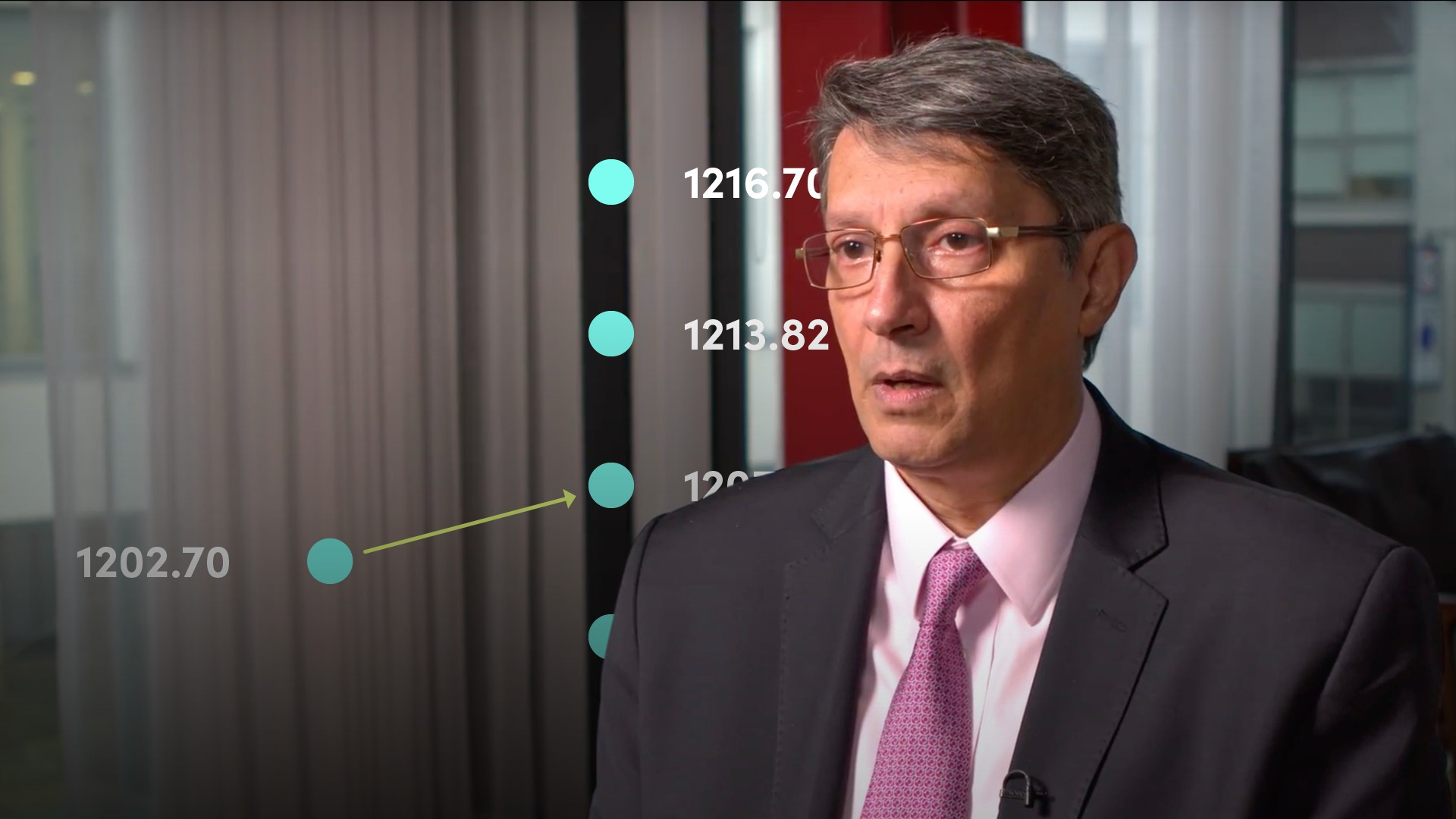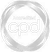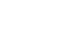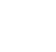# Measures of Dispersion### Abdulla Javeri

In this video, Abdulla covers measures of dispersion to determine how the data is spread around the central point.

Financial MathsIn this video, Abdulla covers measures of dispersion to determine how the data is spread around the central point.

Speak to an expert

Speak to an expert today to access this and all of the content on our platform.### Measures of Dispersion

6 mins 35 secs

Overview

Dispersion essentially explains how the data is spread around the central point.

Key learning objectives:

• Identify the key measures of dispersion

• Define range, variance and standard deviation, and be able to calculate them

• Why using percentage returns is more appropriate than absolute numbers in calculations

## Speak to an expert

Speak to an expert today to access this and all of the content on our platform.

Summary

#### What are the key measures of dispersion?

1. Range
2. Variance
3. Standard Deviation

#### How do we calculate the range?

It’s the difference between the highest and lowest point for any period. It is done in absolute terms, but it also works in percentage terms as a percentage of some starting level.

#### How do we calculate the variance?

This is the average of the squared differences from the mean. Take each data point, subtract the mean and square the result. Add up all the squared numbers, and divide by the number of data points. This is seen in the following formula:#### How do we calculate the standard deviation, and when might it be used?

This is simply the square root of the variance. Standard deviation is a key measure in financial markets as a measure of volatility and thus risk. If it’s a high-risk investment, you would expect the dispersion or volatility to be relatively high.

#### In calculations, why is using percentage returns more appropriate than absolute numbers?

Absolute numbers don’t capture the relative magnitude of the change, however, percentage returns will. For example, if you have a closing price of 100, and the next day’s move is 101, that’s a 1% move. On the other hand, if you have a starting point of 1000 and the next is 1001, this is a 0.1% move. The absolute change is 1, but in relative terms the move from 100 to 101 is substantially larger.

## Speak to an expert

Speak to an expert today to access this and all of the content on our platform.### Abdulla Javeri

Abdulla’s career in the financial markets started in 1990 when he entered the trading floor of the London International Financial Futures Exchange, LIFFE, and qualified as a pit trader in equity and equity index options. In 1996, Abdulla became a trainer for regulatory qualifications and then for non-exam courses, primarily covering all major financial products.

There are no available videos from "Abdulla Javeri"

### Accredited by